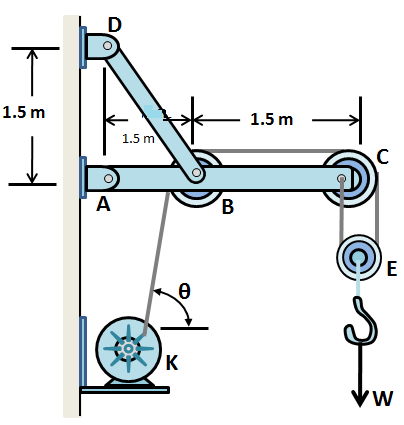# The figure shows a wall crane supporting a load W (=3.0 kN) and \theta =60^{\circ} If the size of...

## Question:

The figure shows a wall crane supporting a load W (=3.0 kN) and {eq}\theta =60^{\circ} {/eq}. If the size of the pulleys is small enough to be neglected, determine the horizontal and vertical components of reaction at pins A and D. Also, what is the force in the cable at the winch K assuming all the pulleys are frictionless? The jib ABC has a weight of 0.5 kN and member BD has a weight of 0.2 kN. Each member is uniform and has a center of gravity at its center.## Resultant Force

The resultant force in any system is given by resolving all forces acting on the body in a single value of force. The effect of the resultant force is the same as the effect of individual force system.

Become a Study.com member to unlock this answer! Create your account

Given data

• Magnitude of load is: {eq}W = 3\;{\rm{kN}} {/eq}
• Angle at K is: {eq}\theta = 60^\circ {/eq}
• Weight of jib ABC is: {eq}{w_1} =...Resultant Force: Definition & Formula

from

Chapter 18 / Lesson 5
42K

In lots of situations, more than one force may act on an object. In this lesson, learn how to find the resultant force of two forces on a single object and how to use this to determine how the object will move.